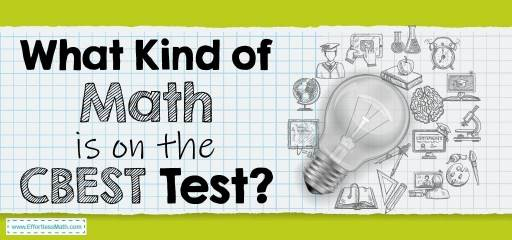# What Kind of Math Is on the CBEST Test?The California Basic Educational Skills Test, known as the CBEST, is required for all potential general education teachers in the state. The CBEST test includes three topics:

• Math
• Writing

Participants have four hours to complete the CBEST test, and there is no time limit for each section. Depending on your local test site, you may take a paper and pencil test or a computer test.

The CBEST math test not only assesses your ability to perform various math functions and answer problems but also knows how to use math knowledge for a set of common learning situations. Many of the questions you find in this test are related to the concepts you learned in high school.

You can expect to find about 50 math questions. All of the math questions will be in multiple-choice format. When it comes to categorizing exam questions, 40% focus on an assortment of nondescript concepts, 20% on geometry, and 40% center around arithmetic. Below we discuss each of the questions asked in the test.

## Numerical and graphical relationships

This section focuses primarily on data – graphs, charts, spreadsheets, tables, and percentages. You must use the data provided to answer the questions. Find out how the two pieces of data relate to each other. Fill in the blanks of the data sheets based on the patterns in the other inputs and understand the different data rules.

## Computation and problem solve

The questions in this section require you to use your knowledge of basic arithmetic functions – division, multiplication, subtraction, and addition. You are also expected to analyze word problems and find answers to them through a technique different from the norm, as well as figure out if you can come to a reasonable answer to a problem based on the data provided.

## Estimation, measurement, and statistical principles

It mainly deals with your ability to evaluate statistical properties and functions, as well as to estimate numerical and measurement data. This section is further divided into two distinct subsets:

### Statistical principles

These sections include probability, percentage, and the average, among other basic elements of statistics. You must also demonstrate your knowledge of standardized examinations and how they are scored. Some questions give you hypothetical grade information that you should use to compare a student’s grades with the rest of the group.

### Estimation and measurement

This section will evaluate your numerical guessing abilities. Questions of subset require you to find the ballpark answer for an arithmetic equation. Find measurements in specific conditions or contexts (like temperature or capacity).

## Is the CBEST Math test hard?

The CBEST math test is not difficult, and you can pass this test with enough study and practice.

## How do I study for the CBEST Math?

How you prepare for the CBEST math test is very important. You can use good prep books to prepare well for the CBEST math test. You can also use online practice tests for further practice after studying the CBEST exam courses.

## How is the CBEST test scored?

You need to earn a minimum score of 41 to pass the mathematics subtest.

## More from Effortless Math for CBEST Test…

### How much do you know about the CBEST test?

What Does the CBEST Test Qualify You For is an article that complements your basic CBEST test information.

### Want to have a study plan for the CBEST math test?

wait! Read our article first: Top 10 Tips to Create a CBEST Math Study Plan

## Have any questions about the CBEST Test?

### What people say about "What Kind of Math Is on the CBEST Test? - Effortless Math: We Help Students Learn to LOVE Mathematics"?

No one replied yet.

X
49% OFF

Limited time only!

Save Over 49%

SAVE \$34

It was \$69.99 now it is \$35.99